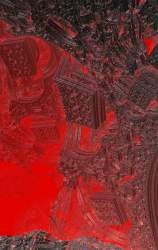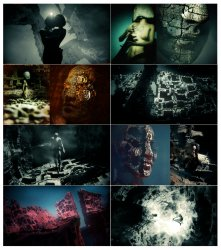News: Check out the originating "3d Mandelbulb" thread here## The All New FractalForums is now in Public Beta Testing! Visit FractalForums.org and check it out!

Where the Dragons of Gold sup freely from the river of Blood & the Mercury mist risesPrevious Image | Next Image
Description: Fragmentarium- Gnarl frag. Luca&Crist

#info gnarl by dark-beam and crist-jroger
#include "MathUtils.frag"
#include "DE-Kn2cr11.frag"

uniform int Giter; slider[0,16,1000]
uniform float Gpow; slider[0,1,3]
uniform vec2 G1; slider[(0,0),(0,0),(2,2)]
uniform float Gheight; slider[0,1,5]
//uniform float Gnarl; slider[0,2,5]
uniform vec2 Gmove; slider[(0,0),(5,5),(20,20)]
uniform vec2 Gnarl; slider[(0,0),(1,1),(3,3)]

float Mag(vec3 pos) {
vec3 z=pos;
int i=0;
float Xold = z.x;
float Yold = z.y;
float xn = 0.0;
float yn = 0.0;
for (i=0; i<Giter; i++) {
xn = z.x - (0.1+G1.x/10)* sin(z.y + Gmove.x*sin(2*z.y-0.2*Gnarl.x*z.x));
yn = z.y - (0.1+G1.y/10)* sin(z.x + Gmove.y*sin(2*z.x-0.2*Gnarl.y*z.y));
z.x= xn; z.y= yn;
}
xn = Xold-z.x;
yn = Yold-z.y;
float mag = 1.5*Gheight + xn*xn+ yn*yn;
mag = log(mag*(Gpow+0.0001)); //smooth
//return z.z-abs(mag);
return z.z-mag;
}

float DE(vec3 pos) {
return pos.z*Mag(pos);
}

#preset default
FOV = 1.35142
Eye = 0.664,42.758,-1.688
Target = -4.93729,17.8773,30.9307
UpLock = false
FocalPlane = 1.80814
Aperture = 0
InFocusAWidth = 0.46845
DofCorrect = false
ApertureNbrSides = 7
ApertureRot = 10
ApStarShaped = false
Gamma = 1.70255
ToneMapping = 5
Exposure = 0.8835
Brightness = 1.03935
Contrast = 1.7738
Saturation = 1.48855
GaussianWeight = 1
AntiAliasScale = 0.4164
Bloom = true
BloomIntensity = 0.9881
BloomPow = 2.1542
BloomTaps = 24
BloomStrong = 6.59759
Detail = -2.00025
RefineSteps = 16
FudgeFactor = 0.58712
MaxRaySteps = 627
MaxDistance = 138.59
Dither = 0.55858
NormalBackStep = 0.8024
DetailAO = -1.00002
coneApertureAO = 0.56998
maxIterAO = 49
FudgeAO = 1
AO_ambient = 1.41086
AO_camlight = 1.09982
AO_pointlight = 0.60072
AoCorrect = 0.44894
Specular = 0.13434
SpecularExp = 272.89
CamLight = 0.760784,0.827451,1,0.16082
AmbiantLight = 1,0.960784,0.815686,0.3516
Reflection = 0.243137,0.243137,0.243137
ReflectionsNumber = 3
SpotGlow = true
SpotLight = 1,0.87451,0.160784,4.1632
LightPos = 6.465,-5.8662,-9.8356
LightSize = 0.08475
LightFallOff = 0.1855
LightGlowExp = 0.447
perf = true
SSS = true
sss1 = 0.17206
sss2 = 0.27945
BaseColor = 1,0.835294,0.631373
OrbitStrength = 0
X = 0.5,0.6,0.6,0.69528
Y = 1,0.6,0,0.52364
Z = 0.8,0.78,1,0.6976
R = 0.490196,0.490196,0.364706,0.96496
BackgroundColor = 0.505882,0.811765,1
CycleColors = true
Cycles = 2.55815
EnableFloor = true
FloorNormal = -0.00248,0,0.84298
FloorHeight = -0.124
FloorColor = 1,0.00784314,0.0235294
HF_Fallof = 5
HF_Const = 0.0551
HF_Intensity = 0.05973
HF_Dir = 0.52358,0.00874,-0.90682
HF_Offset = 0.3958
HF_Color = 0.478431,0.607843,0.619608,1.47768
HF_Scatter = 4.3355
HF_Anisotropy = 0,0,0
HF_FogIter = 6
EnCloudsDir = true NotLocked
Clouds_Dir = -0.23616,-0.14694,-0.19214 NotLocked
CloudScale = 9.30967 NotLocked
CloudFlatness = 0.06186 NotLocked
CloudTops = -9.7496 NotLocked
CloudBase = -9.9522 NotLocked
CloudDensity = 0.23252 NotLocked
CloudRoughness = 1.01086 NotLocked
CloudContrast = 2.1794 NotLocked
CloudColor = 0.698039,0.443137,0.0901961 NotLocked
CloudColor2 = 0.847059,0.0392157,0.188235 NotLocked
SunLightColor = 0.462745,0.698039,0.619608 NotLocked
Cloudvar1 = 0.99 NotLocked
Cloudvar2 = 7.71384 NotLocked
CloudIter = 5 NotLocked
CloudBgMix = 0.64783 NotLocked
Giter = 514
Gpow = 0.1008
G1 = 2,0.3839
Gheight = 0.7226
Gmove = 3.3138,3.979
Gnarl = 1.89504,1.75347
Up = -0.29804,-1.41519,0.0515301
#endpreset

Stats:
Total Favorities: 0 View Who Favorited
Filesize: 302.48kB
Height: 1080 Width: 1920
Discussion Topic: View Topic
Keywords: timemit fragmentarium gnarl gold
Posted by: Tim EmitSeptember 21, 2016, 09:45:45 AM

Rating:by 1 members.

 Sea DragonsRating:Filesize: 852.94kBDate: June 15, 2010, 07:31:10 PMComments (0)By: Ross Hilbert In the blood machineryRating:Filesize: 491.63kBDate: October 19, 2010, 05:50:21 PMComments (0)By: HMN Mandelbulb Crown Flower with Pink MistRating: (None)Filesize: 396.08kBDate: February 10, 2011, 12:31:51 AMComments (0)By: Guest MercuryRating:Filesize: 3.07MBDate: May 10, 2016, 10:14:52 AMComments (1)By: Fractalisman Blood from the air (soft nudity)Rating:Filesize: 2.14MBDate: May 10, 2016, 11:50:30 AMComments (4)By: arias
 Comments (2)Tim Emit ConquerorPosts: 111October 13, 2016, 10:34:49 AMthanks youSabine Fractal FertilizerPosts: 373September 21, 2016, 07:05:03 PMWild canyon!